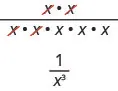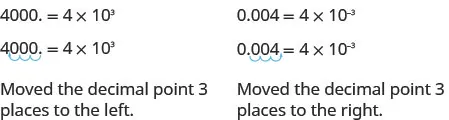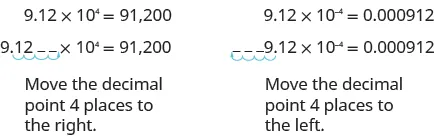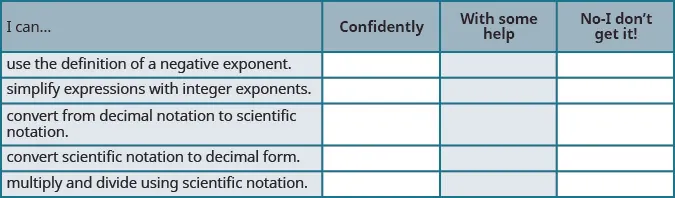Elementary Algebra

# 6.7Integer Exponents and Scientific Notation

Elementary Algebra6.7 Integer Exponents and Scientific Notation

### Learning Objectives

By the end of this section, you will be able to:

• Use the definition of a negative exponent
• Simplify expressions with integer exponents
• Convert from decimal notation to scientific notation
• Convert scientific notation to decimal form
• Multiply and divide using scientific notation

### Be Prepared 6.7

Before you get started, take this readiness quiz.

1. What is the place value of the $66$ in the number $64,89164,891$?
If you missed this problem, review Example 1.1.
2. Name the decimal: $0.0012.0.0012.$
If you missed this problem, review Example 1.91.
3. Subtract: $5−(−3).5−(−3).$
If you missed this problem, review Example 1.42.

### Use the Definition of a Negative Exponent

We saw that the Quotient Property for Exponents introduced earlier in this chapter, has two forms depending on whether the exponent is larger in the numerator or the denominator.

### Quotient Property for Exponents

If $aa$ is a real number, $a≠0a≠0$, and $mandnmandn$ are whole numbers, then

$aman=am−n,m>nandaman=1an−m,n>maman=am−n,m>nandaman=1an−m,n>m$

What if we just subtract exponents regardless of which is larger?

Let’s consider $x2x5x2x5$.

We subtract the exponent in the denominator from the exponent in the numerator.

$x2x5x2−5x−3x2x5x2−5x−3$

We can also simplify $x2x5x2x5$ by dividing out common factors:This implies that $x−3=1x3x−3=1x3$ and it leads us to the definition of a negative exponent.

### Negative Exponent

If $nn$ is an integer and $a≠0a≠0$, then $a−n=1ana−n=1an$.

The negative exponent tells us we can re-write the expression by taking the reciprocal of the base and then changing the sign of the exponent.

Any expression that has negative exponents is not considered to be in simplest form. We will use the definition of a negative exponent and other properties of exponents to write the expression with only positive exponents.

For example, if after simplifying an expression we end up with the expression $x−3x−3$, we will take one more step and write $1x31x3$. The answer is considered to be in simplest form when it has only positive exponents.

### Example 6.89

Simplify: $4−24−2$ $10−3.10−3.$

### Try It 6.177

Simplify: $2−32−3$ $10−7.10−7.$

### Try It 6.178

Simplify: $3−23−2$ $10−4.10−4.$

In Example 6.89 we raised an integer to a negative exponent. What happens when we raise a fraction to a negative exponent? We’ll start by looking at what happens to a fraction whose numerator is one and whose denominator is an integer raised to a negative exponent.

 $1a−n1a−n$ Use the definition of a negative exponent, $a−n=1ana−n=1an$. $11an11an$ Simplify the complex fraction. $1·an11·an1$ Multiply. $anan$

This leads to the Property of Negative Exponents.

### Property of Negative Exponents

If $nn$ is an integer and $a≠0a≠0$, then $1a−n=an1a−n=an$.

### Example 6.90

Simplify: $1y−41y−4$ $13−2.13−2.$

### Try It 6.179

Simplify: $1p−81p−8$ $14−3.14−3.$

### Try It 6.180

Simplify: $1q−71q−7$ $12−4.12−4.$

Suppose now we have a fraction raised to a negative exponent. Let’s use our definition of negative exponents to lead us to a new property.

 $(34)−2(34)−2$ Use the definition of a negative exponent, $a−n=1ana−n=1an$. $1(34)21(34)2$ Simplify the denominator. $19161916$ Simplify the complex fraction. $169169$ But we know that $169169$ is $(43)2(43)2$. This tells us that: $(34)−2=(43)2(34)−2=(43)2$
Table 6.4

To get from the original fraction raised to a negative exponent to the final result, we took the reciprocal of the base—the fraction—and changed the sign of the exponent.

This leads us to the Quotient to a Negative Power Property.

### Quotient to a Negative Exponent Property

If $aandbaandb$ are real numbers, $a≠0,b≠0,a≠0,b≠0,$ and $nn$ is an integer, then $(ab)−n=(ba)n(ab)−n=(ba)n$.

### Example 6.91

Simplify: $(57)−2(57)−2$ $(−2xy)−3.(−2xy)−3.$

### Try It 6.181

Simplify: $(23)−4(23)−4$ $(−6mn)−2.(−6mn)−2.$

### Try It 6.182

Simplify: $(35)−3(35)−3$ $(−a2b)−4.(−a2b)−4.$

When simplifying an expression with exponents, we must be careful to correctly identify the base.

### Example 6.92

Simplify: $(−3)−2(−3)−2$ $−3−2−3−2$ $(−13)−2(−13)−2$ $−(13)−2.−(13)−2.$

### Try It 6.183

Simplify: $(−5)−2(−5)−2$ $−5−2−5−2$ $(−15)−2(−15)−2$ $−(15)−2.−(15)−2.$

### Try It 6.184

Simplify: $(−7)−2(−7)−2$ $−7−2−7−2$, $(−17)−2(−17)−2$ $−(17)−2.−(17)−2.$

We must be careful to follow the Order of Operations. In the next example, parts (a) and (b) look similar, but the results are different.

### Example 6.93

Simplify: $4·2−14·2−1$ $(4·2)−1.(4·2)−1.$

### Try It 6.185

Simplify: $6·3−16·3−1$ $(6·3)−1.(6·3)−1.$

### Try It 6.186

Simplify: $8·2−28·2−2$ $(8·2)−2.(8·2)−2.$

When a variable is raised to a negative exponent, we apply the definition the same way we did with numbers. We will assume all variables are non-zero.

### Example 6.94

Simplify: $x−6x−6$ $(u4)−3.(u4)−3.$

### Try It 6.187

Simplify: $y−7y−7$ $(z3)−5.(z3)−5.$

### Try It 6.188

Simplify: $p−9p−9$ $(q4)−6.(q4)−6.$

When there is a product and an exponent we have to be careful to apply the exponent to the correct quantity. According to the Order of Operations, we simplify expressions in parentheses before applying exponents. We’ll see how this works in the next example.

### Example 6.95

Simplify: $5y−15y−1$ $(5y)−1(5y)−1$ $(−5y)−1.(−5y)−1.$

### Try It 6.189

Simplify: $8p−18p−1$ $(8p)−1(8p)−1$ $(−8p)−1.(−8p)−1.$

### Try It 6.190

Simplify: $11q−111q−1$ $(11q)−1(11q)−1$$−(11q)−1−(11q)−1$ $(−11q)−1.(−11q)−1.$

With negative exponents, the Quotient Rule needs only one form $aman=am−naman=am−n$, for $a≠0a≠0$. When the exponent in the denominator is larger than the exponent in the numerator, the exponent of the quotient will be negative.

### Simplify Expressions with Integer Exponents

All of the exponent properties we developed earlier in the chapter with whole number exponents apply to integer exponents, too. We restate them here for reference.

### Summary of Exponent Properties

If $aandbaandb$ are real numbers, and $mandnmandn$ are integers, then

$Product Propertyam·an=am+nPower Property(am)n=am·nProduct to a Power(ab)m=ambmQuotient Propertyaman=am−n,a≠0Zero Exponent Propertya0=1,a≠0Quotient to a Power Property(ab)m=ambm,b≠0Properties of Negative Exponentsa−n=1anand1a−n=anQuotient to a Negative Exponent(ab)−n=(ba)nProduct Propertyam·an=am+nPower Property(am)n=am·nProduct to a Power(ab)m=ambmQuotient Propertyaman=am−n,a≠0Zero Exponent Propertya0=1,a≠0Quotient to a Power Property(ab)m=ambm,b≠0Properties of Negative Exponentsa−n=1anand1a−n=anQuotient to a Negative Exponent(ab)−n=(ba)n$

### Example 6.96

Simplify: $x−4·x6x−4·x6$ $y−6·y4y−6·y4$ $z−5·z−3.z−5·z−3.$

### Try It 6.191

Simplify: $x−3·x7x−3·x7$ $y−7·y2y−7·y2$ $z−4·z−5.z−4·z−5.$

### Try It 6.192

Simplify: $a−1·a6a−1·a6$ $b−8·b4b−8·b4$ $c−8·c−7.c−8·c−7.$

In the next two examples, we’ll start by using the Commutative Property to group the same variables together. This makes it easier to identify the like bases before using the Product Property.

### Example 6.97

Simplify: $(m4n−3)(m−5n−2).(m4n−3)(m−5n−2).$

### Try It 6.193

Simplify: $(p6q−2)(p−9q−1).(p6q−2)(p−9q−1).$

### Try It 6.194

Simplify: $(r5s−3)(r−7s−5).(r5s−3)(r−7s−5).$

If the monomials have numerical coefficients, we multiply the coefficients, just like we did earlier.

### Example 6.98

Simplify: $(2x−6y8)(−5x5y−3).(2x−6y8)(−5x5y−3).$

### Try It 6.195

Simplify: $(3u−5v7)(−4u4v−2).(3u−5v7)(−4u4v−2).$

### Try It 6.196

Simplify: $(−6c−6d4)(−5c−2d−1).(−6c−6d4)(−5c−2d−1).$

In the next two examples, we’ll use the Power Property and the Product to a Power Property.

### Example 6.99

Simplify: $(6k3)−2.(6k3)−2.$

### Try It 6.197

Simplify: $(−4x4)−2.(−4x4)−2.$

### Try It 6.198

Simplify: $(2b3)−4.(2b3)−4.$

### Example 6.100

Simplify: $(5x−3)2.(5x−3)2.$

### Try It 6.199

Simplify: $(8a−4)2.(8a−4)2.$

### Try It 6.200

Simplify: $(2c−4)3.(2c−4)3.$

To simplify a fraction, we use the Quotient Property and subtract the exponents.

### Example 6.101

Simplify: $r5r−4.r5r−4.$

### Try It 6.201

Simplify: $x8x−3.x8x−3.$

### Try It 6.202

Simplify: $y8y−6.y8y−6.$

### Convert from Decimal Notation to Scientific Notation

Remember working with place value for whole numbers and decimals? Our number system is based on powers of 10. We use tens, hundreds, thousands, and so on. Our decimal numbers are also based on powers of tens—tenths, hundredths, thousandths, and so on. Consider the numbers 4,000 and $0.0040.004$. We know that 4,000 means $4×1,0004×1,000$ and 0.004 means $4×11,0004×11,000$.

If we write the 1000 as a power of ten in exponential form, we can rewrite these numbers in this way:

$4,0000.0044×1,0004×11,0004×1034×11034×10−34,0000.0044×1,0004×11,0004×1034×11034×10−3$

When a number is written as a product of two numbers, where the first factor is a number greater than or equal to one but less than 10, and the second factor is a power of 10 written in exponential form, it is said to be in scientific notation.

### Scientific Notation

A number is expressed in scientific notation when it is of the form

It is customary in scientific notation to use as the $××$ multiplication sign, even though we avoid using this sign elsewhere in algebra.

If we look at what happened to the decimal point, we can see a method to easily convert from decimal notation to scientific notation.In both cases, the decimal was moved 3 places to get the first factor between 1 and 10.

$The power of 10 is positive when the number is larger than 1:4,000=4×103The power of 10 is negative when the number is between 0 and 1:0.004=4×10−3The power of 10 is positive when the number is larger than 1:4,000=4×103The power of 10 is negative when the number is between 0 and 1:0.004=4×10−3$

### Example 6.102

#### How to Convert from Decimal Notation to Scientific Notation

Write in scientific notation: 37,000.

### Try It 6.203

Write in scientific notation: $96,000.96,000.$

### Try It 6.204

Write in scientific notation: $48,300.48,300.$

### How To

#### Convert from decimal notation to scientific notation

1. Step 1. Move the decimal point so that the first factor is greater than or equal to 1 but less than 10.
2. Step 2. Count the number of decimal places, n, that the decimal point was moved.
3. Step 3.
Write the number as a product with a power of 10.
If the original number is:
• greater than 1, the power of 10 will be 10n.
• between 0 and 1, the power of 10 will be 10−n.
4. Step 4. Check.

### Example 6.103

Write in scientific notation: $0.0052.0.0052.$

### Try It 6.205

Write in scientific notation: $0.0078.0.0078.$

### Try It 6.206

Write in scientific notation: $0.0129.0.0129.$

### Convert Scientific Notation to Decimal Form

How can we convert from scientific notation to decimal form? Let’s look at two numbers written in scientific notation and see.

$9.12×1049.12×10−49.12×10,0009.12×0.000191,2000.0009129.12×1049.12×10−49.12×10,0009.12×0.000191,2000.000912$

If we look at the location of the decimal point, we can see an easy method to convert a number from scientific notation to decimal form.

$9.12×104=91,2009.12×10−4=0.0009129.12×104=91,2009.12×10−4=0.000912$In both cases the decimal point moved 4 places. When the exponent was positive, the decimal moved to the right. When the exponent was negative, the decimal point moved to the left.

### Example 6.104

#### How to Convert Scientific Notation to Decimal Form

Convert to decimal form: $6.2×103.6.2×103.$

### Try It 6.207

Convert to decimal form: $1.3×103.1.3×103.$

### Try It 6.208

Convert to decimal form: $9.25×104.9.25×104.$

The steps are summarized below.

### How To

#### Convert scientific notation to decimal form.

To convert scientific notation to decimal form:

1. Step 1. Determine the exponent, $nn$, on the factor 10.
2. Step 2.
Move the decimal $nn$ places, adding zeros if needed.
• If the exponent is positive, move the decimal point $nn$ places to the right.
• If the exponent is negative, move the decimal point $|n||n|$ places to the left.
3. Step 3. Check.

### Example 6.105

Convert to decimal form: $8.9×10−2.8.9×10−2.$

### Try It 6.209

Convert to decimal form: $1.2×10−4.1.2×10−4.$

### Try It 6.210

Convert to decimal form: $7.5×10−2.7.5×10−2.$

### Multiply and Divide Using Scientific Notation

Astronomers use very large numbers to describe distances in the universe and ages of stars and planets. Chemists use very small numbers to describe the size of an atom or the charge on an electron. When scientists perform calculations with very large or very small numbers, they use scientific notation. Scientific notation provides a way for the calculations to be done without writing a lot of zeros. We will see how the Properties of Exponents are used to multiply and divide numbers in scientific notation.

### Example 6.106

Multiply. Write answers in decimal form: $(4×105)(2×10−7).(4×105)(2×10−7).$

### Try It 6.211

Multiply $(3×106)(2×10−8)(3×106)(2×10−8)$. Write answers in decimal form.

### Try It 6.212

Multiply $(3×10−2)(3×10−1)(3×10−2)(3×10−1)$. Write answers in decimal form.

### Example 6.107

Divide. Write answers in decimal form: $9×1033×10−2.9×1033×10−2.$

### Try It 6.213

Divide $8×1042×10−18×1042×10−1$. Write answers in decimal form.

### Try It 6.214

Divide $8×1024×10−28×1024×10−2$. Write answers in decimal form.

### Media

Access these online resources for additional instruction and practice with integer exponents and scientific notation:

### Section 6.7 Exercises

#### Practice Makes Perfect

Use the Definition of a Negative Exponent

In the following exercises, simplify.

500.

$4−24−2$
$10−310−3$

501.

$3−43−4$
$10−210−2$

502.

$5−35−3$
$10−510−5$

503.

$2−82−8$
$10−210−2$

504.

$1c−51c−5$
$13−213−2$

505.

$1c−51c−5$
$15−215−2$

506.

$1q−101q−10$
$110−3110−3$

507.

$1t−91t−9$
$110−4110−4$

508.

$(58)−2(58)−2$
$(−3mn)−2(−3mn)−2$

509.

$(310)−2(310)−2$
$(−2cd)−3(−2cd)−3$

510.

$(49)−3(49)−3$
$(−u22v)−5(−u22v)−5$

511.

$(72)−3(72)−3$
$(−3xy2)−3(−3xy2)−3$

512.

$(−5)−2(−5)−2$
$−5−2−5−2$
$(−15)−2(−15)−2$
$−(15)−2−(15)−2$

513.

$(−7)−2(−7)−2$
$−7−2−7−2$
$(−17)−2(−17)−2$
$−(17)−2−(17)−2$

514.

$−3−3−3−3$
$(−13)−3(−13)−3$
$−(13)−3−(13)−3$
$(−3)−3(−3)−3$

515.

$−5−3−5−3$
$(−15)−3(−15)−3$
$−(15)−3−(15)−3$
$(−5)−3(−5)−3$

516.

$3·5−13·5−1$
$(3·5)−1(3·5)−1$

517.

$2·5−12·5−1$
$(2·5)−1(2·5)−1$

518.

$4·5−24·5−2$
$(4·5)−2(4·5)−2$

519.

$3·4−23·4−2$
$(3·4)−2(3·4)−2$

520.

$m−4m−4$
$(x3)−4(x3)−4$

521.

$b−5b−5$
$(k2)−5(k2)−5$

522.

$p−10p−10$
$(q6)−8(q6)−8$

523.

$s−8s−8$
$(a9)−10(a9)−10$

524.

$7n−17n−1$
$(7n)−1(7n)−1$
$(−7n)−1(−7n)−1$

525.

$6r−16r−1$
$(6r)−1(6r)−1$
$(−6r)−1(−6r)−1$

526.

$(3p)−2(3p)−2$
$3p−23p−2$
$−3p−2−3p−2$

527.

$(2q)−4(2q)−4$
$2q−42q−4$
$−2q−4−2q−4$

Simplify Expressions with Integer Exponents

In the following exercises, simplify.

528.

$b4b−8b4b−8$
$r−2r5r−2r5$
$x−7x−3x−7x−3$

529.

$s3·s−7s3·s−7$
$q−8·q3q−8·q3$
$y−2·y−5y−2·y−5$

530.

$a3·a−3a3·a−3$
$a·a3a·a3$
$a·a−3a·a−3$

531.

$y5·y−5y5·y−5$
$y·y5y·y5$
$y·y−5y·y−5$

532.

$p 5 · p −2 · p −4 p 5 · p −2 · p −4$

533.

$x 4 · x −2 · x −3 x 4 · x −2 · x −3$

534.

$( w 4 x −5 ) ( w −2 x −4 ) ( w 4 x −5 ) ( w −2 x −4 )$

535.

$( m 3 n −3 ) ( m −5 n −1 ) ( m 3 n −3 ) ( m −5 n −1 )$

536.

$( u v −2 ) ( u −5 v −3 ) ( u v −2 ) ( u −5 v −3 )$

537.

$( p q −4 ) ( p −6 q −3 ) ( p q −4 ) ( p −6 q −3 )$

538.

$( −6 c −3 d 9 ) ( 2 c 4 d −5 ) ( −6 c −3 d 9 ) ( 2 c 4 d −5 )$

539.

$( −2 j −5 k 8 ) ( 7 j 2 k −3 ) ( −2 j −5 k 8 ) ( 7 j 2 k −3 )$

540.

$( −4 r −2 s −8 ) ( 9 r 4 s 3 ) ( −4 r −2 s −8 ) ( 9 r 4 s 3 )$

541.

$( −5 m 4 n 6 ) ( 8 m −5 n −3 ) ( −5 m 4 n 6 ) ( 8 m −5 n −3 )$

542.

$( 5 x 2 ) −2 ( 5 x 2 ) −2$

543.

$( 4 y 3 ) −3 ( 4 y 3 ) −3$

544.

$( 3 z −3 ) 2 ( 3 z −3 ) 2$

545.

$( 2 p −5 ) 2 ( 2 p −5 ) 2$

546.

$t 9 t −3 t 9 t −3$

547.

$n 5 n −2 n 5 n −2$

548.

$x −7 x −3 x −7 x −3$

549.

$y −5 y −10 y −5 y −10$

Convert from Decimal Notation to Scientific Notation

In the following exercises, write each number in scientific notation.

550.

57,000

551.

340,000

552.

8,750,000

553.

1,290,000

554.

0.026

555.

0.041

556.

0.00000871

557.

0.00000103

Convert Scientific Notation to Decimal Form

In the following exercises, convert each number to decimal form.

558.

$5.2 × 10 2 5.2 × 10 2$

559.

$8.3 × 10 2 8.3 × 10 2$

560.

$7.5 × 10 6 7.5 × 10 6$

561.

$1.6 × 10 10 1.6 × 10 10$

562.

$2.5 × 10 −2 2.5 × 10 −2$

563.

$3.8 × 10 −2 3.8 × 10 −2$

564.

$4.13 × 10 −5 4.13 × 10 −5$

565.

$1.93 × 10 −5 1.93 × 10 −5$

Multiply and Divide Using Scientific Notation

566.

$( 3 × 10 −5 ) ( 3 × 10 9 ) ( 3 × 10 −5 ) ( 3 × 10 9 )$

567.

$( 2 × 10 2 ) ( 1 × 10 −4 ) ( 2 × 10 2 ) ( 1 × 10 −4 )$

568.

$( 7.1 × 10 −2 ) ( 2.4 × 10 −4 ) ( 7.1 × 10 −2 ) ( 2.4 × 10 −4 )$

569.

$( 3.5 × 10 −4 ) ( 1.6 × 10 −2 ) ( 3.5 × 10 −4 ) ( 1.6 × 10 −2 )$

570.

$7 × 10 −3 1 × 10 −7 7 × 10 −3 1 × 10 −7$

571.

$5 × 10 −2 1 × 10 −10 5 × 10 −2 1 × 10 −10$

572.

$6 × 10 4 3 × 10 −2 6 × 10 4 3 × 10 −2$

573.

$8 × 10 6 4 × 10 −1 8 × 10 6 4 × 10 −1$

#### Everyday Math

574.

The population of the United States on July 4, 2010 was almost 310,000,000. Write the number in scientific notation.

575.

The population of the world on July 4, 2010 was more than 6,850,000,000. Write the number in scientific notation

576.

The average width of a human hair is 0.0018 centimeters. Write the number in scientific notation.

577.

The probability of winning the 2010 Megamillions lottery was about 0.0000000057. Write the number in scientific notation.

578.

In 2010, the number of Facebook users each day who changed their status to ‘engaged’ was $2×1042×104$. Convert this number to decimal form.

579.

At the start of 2012, the US federal budget had a deficit of more than $1.5×10131.5×1013$. Convert this number to decimal form.

580.

The concentration of carbon dioxide in the atmosphere is $3.9×10−43.9×10−4$. Convert this number to decimal form.

581.

The width of a proton is $1×10−51×10−5$ of the width of an atom. Convert this number to decimal form.

582.

Health care costs The Centers for Medicare and Medicaid projects that consumers will spend more than $4 trillion on health care by 2017. 1. Write 4 trillion in decimal notation. 2. Write 4 trillion in scientific notation. 583. Coin production In 1942, the U.S. Mint produced 154,500,000 nickels. Write 154,500,000 in scientific notation. 584. Distance The distance between Earth and one of the brightest stars in the night star is 33.7 light years. One light year is about 6,000,000,000,000 (6 trillion), miles. 1. Write the number of miles in one light year in scientific notation. 2. Use scientific notation to find the distance between Earth and the star in miles. Write the answer in scientific notation. 585. Debt At the end of fiscal year 2015 the gross United States federal government debt was estimated to be approximately$18,600,000,000,000 (\$18.6 trillion), according to the Federal Budget. The population of the United States was approximately 300,000,000 people at the end of fiscal year 2015.

1. Write the debt in scientific notation.
2. Write the population in scientific notation.
3. Find the amount of debt per person by using scientific notation to divide the debt by the population. Write the answer in scientific notation.

#### Writing Exercises

586.
1. Explain the meaning of the exponent in the expression $2323$.
2. Explain the meaning of the exponent in the expression $2−32−3$.
587.

When you convert a number from decimal notation to scientific notation, how do you know if the exponent will be positive or negative?

#### Self Check

After completing the exercises, use this checklist to evaluate your mastery of the objectives of this section.Overall, after looking at the checklist, do you think you are well-prepared for the next section? Why or why not?

Order a print copy

As an Amazon Associate we earn from qualifying purchases.